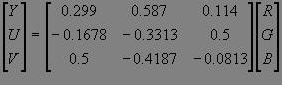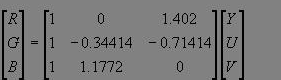# 1 YUV格式与RGB格式说明

由于不同国家的电视信号系统支持的图像格式不同，有YUV格式成像，也有RGB格式成像，因此为了保证兼容性，需要进行RGBYUV格式的互转。

## 2 RGB格式转为YUV格式转换公式将上述矩阵乘法展开，YUV(256 级别可以从8位 RGB 直接计算如下：

Y = 0.299 R + 0.587 G + 0.114 B

U = - 0.1687 R - 0.3313 G + 0.5 B + 128

V = 0.5 R - 0.4187 G - 0.0813 B + 128

## 3 YUV格式转为RGB格式转换公式将上述矩阵乘法展开，8RGB可以从YUV(256级别) 直接计算如下：

R = Y + 1.402 (V-128)

G = Y - 0.34414 (U-128) - 0.71414 (V-128)

B = Y + 1.772 (U-128)

## 4 RGB格式转为YCbCr格式转换公式

YCbCr格式是基于YUV格式的一个偏移，具体转换如下：

Y’ = 0.257*R' + 0.504*G' + 0.098*B' + 16
Cb' = -0.148*R' - 0.291*G' + 0.439*B' + 128
Cr' = 0.439*R' - 0.368*G' - 0.071*B' + 128

## 5 RGB格式转为YCbCr格式转换公式

转换格式如下：

R' = 1.164*(Y-16) + 1.596*(Cr'-128)
G' = 1.164*(Y’-16) - 0.813*(Cr'-128) -0.392*(Cb'-128)

B' = 1.164*(Y’-16) + 2.017*(Cb'-128)©️2019 CSDN 皮肤主题: 大白 设计师: CSDN官方博客